About sedimentation for centrifugal separator| Maker of the separator Saito separator limited

About sedimentation for centrifugal separator| Maker of the separator Saito separator limited

# About sedimentation for centrifugal separator

Everybody has experienced the phenomenon that a certain kind of leaving a mixed solution in a container for a while and finding it separates to several layers with different specific gravities.
Figure 1 shows a model that uses this sedimentation separation phenomenon to create conditions for enabling a continuous separating processes.
In Figure 1, liquid with small suspended particles is flowing in at flow rate Q from point A of a tank with length L, width W, and arbitrary depth, and is steadily flowing out of point B with liquid depth h.
Small particle P flowing in from point A starts settling downward at velocity Vq caused by specific gravity difference and, at the same time, it starts moving at velocity Vg in a horizontal direction with the flow of liquid. Actually, P moves at velocity V in the direction of the sum of Vg and Vq.
Now, to obtain clarified liquid by removing suspended small particle P, P must be settled below liquid depth h before it reaches the flow-out point B. Therefore, separation is enabled if equation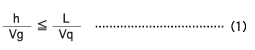is satisfied. Because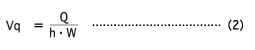is considered correct, (2) may be assigned to (1). Thus,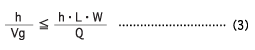will be obtained, where L･W in the right side of equation (3) is the plane area of the tank shown in Figure 1. When the tank plane area is S, equation (3) can be expressed as shown below.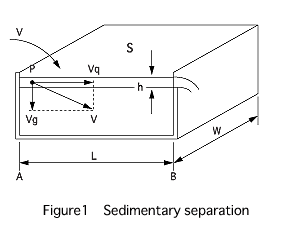This shows that the separative power Q of this tank is determined by settling velocity Vg of small particle P and area S. Vg in this equation is called the plain settling velocity. Stokes has brought the equation shown on the left.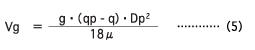The equation on the right side shows that Vg becomes greater in proportion with gravitational acceleration g.
In centrifugal separators (centrifugal sedimentation machines), the settling velocity is increased by centrifugal acceleration instead of gravitational acceleration g to attain the purpose of treating a large quantity in a short period of time. On the other hand, many improvements have been made on the rotating container to increase the area equivalent to S in equation (4).
The capacity of a centrifugal separator is indicated by the centrifugal settling separation area (_-Value) obtained by the rotating container (called rotary drum) structure and the centrifugal acceleration of the plain sedimentation tank assumed from the centrifugal separator.